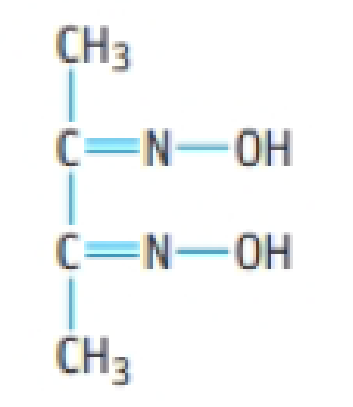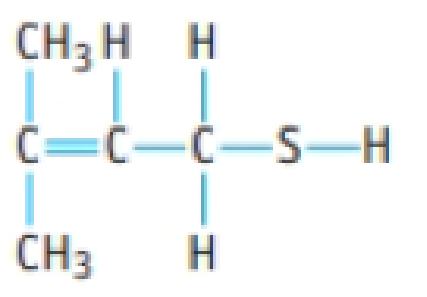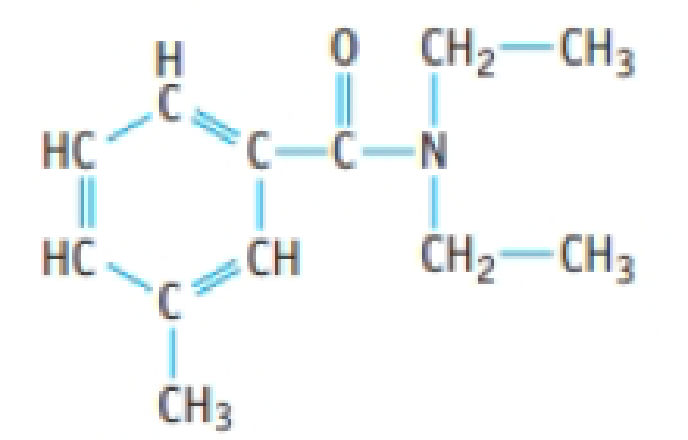# Assume you have 0.123 mol of each of the following compounds. What mass of each is present? (a) C 14 H 10 O 4 , benzoyl peroxide, used in acne medications (b) Dimethylglyoxime, used in the laboratory to test for nickel(II) ions (c) The compound below, responsible for the "skunky" taste in poorly made beer. (d) DEFT, a mosquito repellent### Chemistry & Chemical Reactivity

9th Edition
John C. Kotz + 3 others
Publisher: Cengage Learning
ISBN: 9781133949640

#### Solutions

Chapter
Section### Chemistry & Chemical Reactivity

9th Edition
John C. Kotz + 3 others
Publisher: Cengage Learning
ISBN: 9781133949640
Chapter 2, Problem 74PS
Textbook Problem
74 views

## Assume you have 0.123 mol of each of the following compounds. What mass of each is present?(a) C14H10O4, benzoyl peroxide, used in acne medications(b) Dimethylglyoxime, used in the laboratory to test for nickel(II) ions(c) The compound below, responsible for the "skunky" taste in poorly made beer.(d) DEFT, a mosquito repellent(a)

Interpretation Introduction

Interpretation:

For the given amounts of molecules in moles, the mass conversions are needed to be calculated.

Concept introduction:

Molar mass of benzoyl peroxide, dimethylglyoxime, unnamed compound and DEET are 242.23, 116.12, 104.21 and 191.27.

• Conversion formula for mass of an atom into number moles,

Numberofmoles×Molarmass=Massingrams

### Explanation of Solution

The amount of benzoyl peroxide is given as 0.123mol.

Molar mass of benzoyl peroxide is 242.23g/mol.

Conversion formula for number moles of an atom into mass in grams,

Numberofmoles×Molarma

(b)

Interpretation Introduction

Interpretation:

For the given amounts of molecules in moles, the mass conversions are needed to be calculated.

Concept introduction:

Molar mass of benzoyl peroxide, dimethylglyoxime, unnamed compound and DEET are 242.23, 116.12, 104.21 and 191.27.

• Conversion formula for mass of an atom into number moles,

Numberofmoles×Molarmass=Massingrams

(c)

Interpretation Introduction

Interpretation:

For the given amounts of molecules in moles, the mass conversions are needed to be calculated.

Concept introduction:

Molar mass of benzoyl peroxide, dimethylglyoxime, unnamed compound and DEET are 242.23, 116.12, 104.21 and 191.27.

• Conversion formula for mass of an atom into number moles,

Numberofmoles×Molarmass=Massingrams

(d)

Interpretation Introduction

Interpretation:

For the given amounts of molecules in moles, the mass conversions are needed to be calculated.

Concept introduction:

Molar mass of benzoyl peroxide, dimethylglyoxime, unnamed compound and DEET are 242.23, 116.12, 104.21 and 191.27.

• Conversion formula for mass of an atom into number moles,

Numberofmoles×Molarmass=Massingrams

### Still sussing out bartleby?

Check out a sample textbook solution.

See a sample solution

#### The Solution to Your Study Problems

Bartleby provides explanations to thousands of textbook problems written by our experts, many with advanced degrees!

Get Started

Find more solutions based on key concepts
Define the terms matter and mass. What is the difference between mass and weight?

General Chemistry - Standalone book (MindTap Course List)

This class will give you the skills to learn how to separate legitimate nutrition claims from those that are qu...

Nutrition: Concepts and Controversies - Standalone book (MindTap Course List)

What evidence do scientists have that life on Earth began in the sea?

Foundations of Astronomy (MindTap Course List)

Two spheres having masses M and 2M and radii R and 3R, respectively, are simultaneously released from rest when...

Physics for Scientists and Engineers, Technology Update (No access codes included)

Where are distinct water masses formed?

Oceanography: An Invitation To Marine Science, Loose-leaf Versin